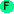# Conditionals

The fundamental conditional operator in Arc is `if`. It is similar to the Lisp `if`, except it eliminates the parentheses around the clauses. For a simple conditional with multiple body statements, `when` or its opposite `unless` can be used.

Arc provides several conditionals that assign the test expression to a variable, similar to `let`. The `iflet`, `caselet`, and `whenlet` macros are useful if the test expression is used inside the body.

## Conditionalsif [test expr] ... [else-expr] Arc is the basic conditional operation. It takes a sequence of tests and expressions. The expression corresponding to the first true test is returned. Other expressions are not evaluated. ```>(if nil "Nil is true" 0 "0 is true" "What is true?") "0 is true" ```iflet var expr then-expr [test1 expr1] ... [else-expr] Evaluates `expr`. If true, `expr` is assigned to `var` and `then-expr` is evaluated and returned. Otherwise, the remaining arguments are processed as normal `if` clauses. ```>(iflet x 42 (+ x 1)) 43 ``` ```>(iflet x nil (+ x 1)) nil ``` ```>(iflet x nil (+ x 1) (< 1 2) 55) 55 ```when test [body ...] Executes body if test is true. This is similar to `if`, except it allows multiple body statements but only has a single test clause. ```>(when 1 (pr "a") (pr "b")) ab "b" ```whenlet var expr [body ...] Evaluates `expr`. If true, the value is assigned to `var` and `body` is executed. ```>(whenlet x nil (prn "hi") (+ x 1)) nil ``` ```>(whenlet x 1 (prn "hi") (+ x 1)) hi 2 ```unless test [body ...] Executes body if test is false. This is the opposite of 'when'. ```>(unless 1 (pr "a") (pr "b")) nil ```case arg [test1 expr1] ... [else-expr] `arg` is evaluated. It is then compared to the test values in sequence. If it matches one, the corresponding expr is evaluated and returned. If there is no match and no `else-expr`, `nil` is returned. ```>(case 'b a 1 b 2 3) 2 ``` ```>(case 42 10 "foo" 42 "bar") "bar" ```caselet var arg [test1 expr1] ... [else-expr] `arg` is evaluated and assigned to `var`. It is then compared to the test values in sequence. If it matches one, the corresponding expr is evaluated and returned. If there is no match and no `else-expr`, `nil` is returned. ```>(caselet x 'b a 1 b 2 3) 2 ``` ```>(caselet x 42 10 (+ x 3) 42 (+ x 5)) 47 ```check expr test [alt] Evaluates `expr` and applies predicate `test`. If true, returns the evaluated value. Otherwise returns `alt` or nil. `alt` can be a function to try again, even calling `check` recursively. ```>(check (+ 10 10) odd "foo") "foo" ``` ```>(check (+ 10 10) even "bar") 20 ```